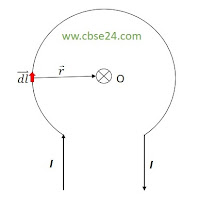# Magnetic Field at the Centre of Circular Current Loop | cbse24

Consider a circular loop of wire of radius r carrying current I .We wish to calculate its magnetic field at the center O. The entire loop can be divided into a large number of small current elements.Magnetic field at the center of a circular current loop

Consider a current element dl of the loop. According to Bio-Severt law, the magnetic field at the center O due to this element is

The field at point O points normally into the plane of paper, as shown by enclosed cross ⊗.The direction of dl is along the tangent, so dlr . Consequently, the magnetic field at the center O due to this current element is

*